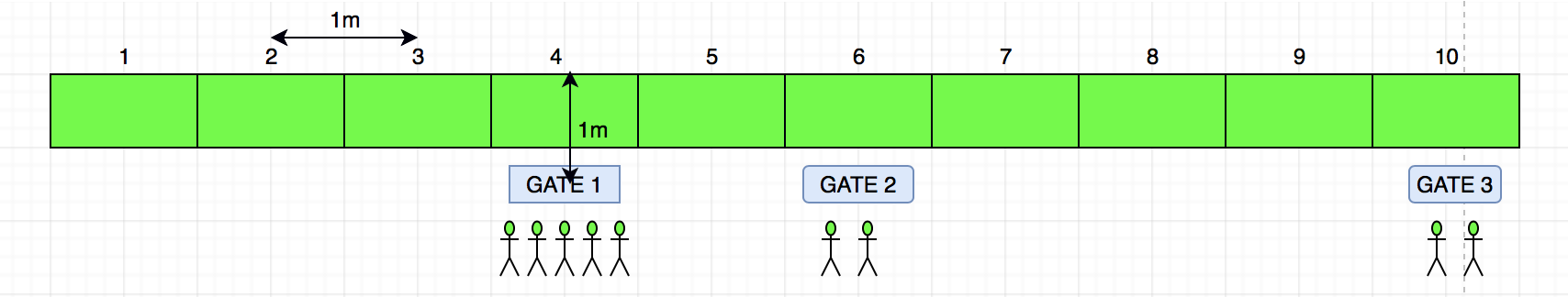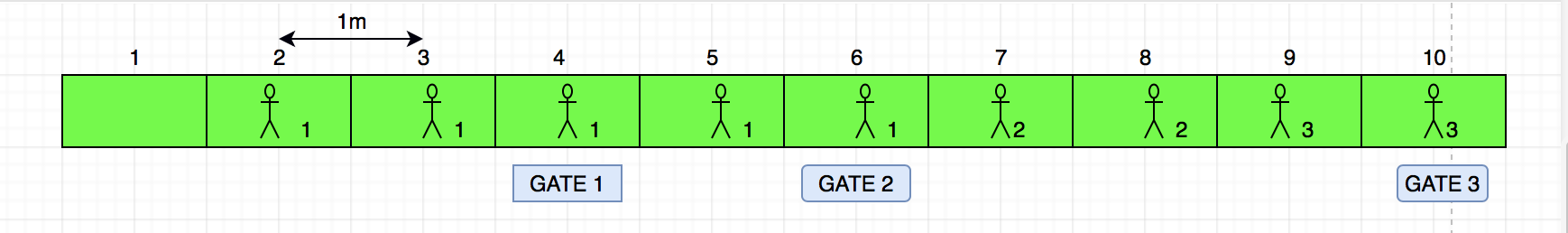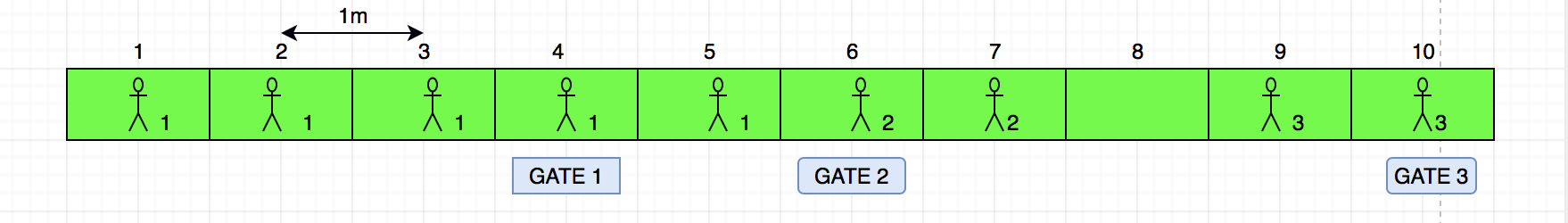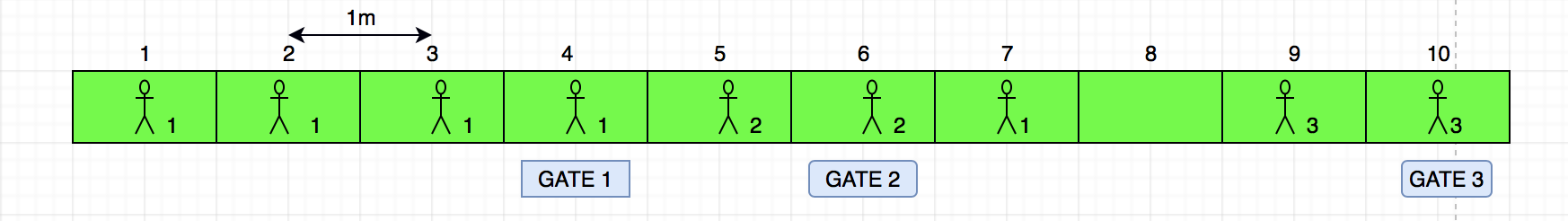# Samsung Semiconductor Institute of Research (SSIR software) intern/FTE | Set-1

• Difficulty Level : Hard
• Last Updated : 04 Aug, 2021

There are N fishing spots and 3 gates. At each gate there are some fishermen waiting to reach the nearest unoccupied fishing spot. (Total no of fisherman <=N)
Distance between consecutive spots = distance between gate and nearest spot = 1 m
Only 1 gate can be opened at a time and all fishermen of that gate must occupy the spots before the next gate is opened.
Distance is calculated as gate to nearest spot + nearest spot to closest vacant spot.
Find the total sum of minimum distances need to walk for all the fishermen.
Inputs to be taken:
Number of fishing spots
Position of the gates
Number of fishermen at each gatesTotal number of fishing spots= 10
5 fisherman at gate 1 located at position 4,
2 fishermen at gate 2 located at position 6,
2 fishermen at gate 3 located at position 10,
If gates are opened in order G1->G2->G3

The arrangement will be:Distance is calculated as gate to nearest spot + nearest spot to closest vacant spot.
After G1 gate is opened, fishermen are placed at spots marked with 1.
Distance = 11m
After G2 gate is opened, fishermen are placed at spots marked with 2.
Distance = 5m
After G3 gate is opened, fishermen are placed at spots marked with 3.
Distance = 3m
Total distance in this order : 11 + 5 + 3 = 19

If gates are opened in order G2->G1->G3
Case1 – Last fisherman of gate 2 is placed at position 7
the final arrangement will be:After G2 gate is opened, fishermen are placed at spots marked with 2.
Distance = 3m
After G1 gate is opened, fishermen are placed at spots marked with 1.
Distance = 12m
After G3 gate is opened, fishermen are placed at spots marked with 3.
Distance = 3m
Total distance in this order : 3+12+3 = 18

Case2 – Last fisherman of gate 2 is placed at position 5
the final arrangement will be:After G2 gate is opened, fishermen are placed at spots marked with 2.
Distance = 3m
After G1 gate is opened, fishermen are placed at spots marked with 1.
Distance = 14m
After G3 gate is opened, fishermen are placed at spots marked with 3.
Distance = 3m
Total distance in this order : 3+14+3 = 20
Other cases are redundant
so minimum distance is 18
Solution:

Generate all combinations and assigns fishermen in all gate combinations to calculate minimum walking distance.
Generating combination can be done in both recursive and iterative way. Now to eliminate unnecessary permutations we can conclude that, for minimum walking distances, fishermen from a particular gate must stay together i.e they should occupy consecutive fishing spots. Thus we can visualise the problem as 3 blocks(group of fisherman) sliding through the entire fishing range. The minimum of which is the answer.

## C++

 `#define _CRT_SECURE_NO_WARNINGS``#include``using` `namespace` `std;``#define MAX 3` `int` `fishspot; ``// fishing spots``int` `gate[MAX]; ``// position of gates``int` `fishermen[MAX]; ``// no of fishermen at each gate``int` `N; ``// total no of fishing spots``int` `visited[MAX]; ``// occupied fishing spots``int` `Answer; ``// result` `// To unmark spots occupied by fishermen of gate# index``class` `GFG``{``public` `:``void` `reset_fishspot(``int` `index)``{``    ``int` `i;``    ``for` `(i = 1; i <= N; i++)``        ``if` `(fishspot[i] == index + 1)``            ``fishspot[i] = 0;``}` `// Calculate minimum distance while``// allotting spots to fishermen of gate# index.``// Returns number of positions possible``// with minimum distance.``// pos1, pos2 is used to return positions``int` `calculate_distance(``int` `index, ``int``*pos1,``                    ``int` `*pos2, ``int` `*score)``{``    ``int` `i, sum = 0, left_min = 999999,``                    ``right_min = 999999,``                    ``left, right, npos = 0;``    ``*pos1 = *pos2 = *score = 0;` `    ``left = right = gate[index];` `    ``// Allot spots to all fishermen except``    ``// last based on minimum distance``    ``for` `(i = 1; i < fishermen[index]; i++)``    ``{``        ``if` `(fishspot[gate[index]] == 0)``        ``{``            ``sum++;``            ``fishspot[gate[index]] = index + 1;``        ``}``        ``else``        ``{``            ``left_min = right_min = 999999;` `            ``while` `((left > 0) && (fishspot[left] > 0))``                ``left--;` `            ``while` `((right <= N) &&``                ``(fishspot[right] > 0))``                ``right++;` `            ``if` `((left > 0) && (fishspot[left] == 0))``                ``left_min = gate[index] - left + 1;` `            ``if` `((right <= N) && (fishspot[right] == 0))``                ``right_min = right - gate[index] + 1;` `            ``if` `(right_min == left_min)``            ``{``                ``// Place 2 fishermen, if available``                ``if` `((fishermen[index] - i - 1) > 1)``                ``{``                    ``fishspot[left] = fishspot[right] = index + 1;``                    ``sum += (2 * left_min);``                    ``i++;` `                    ``// If all fishermen are alloted spots``                    ``if` `(i == fishermen[index])``                    ``{``                        ``npos = 1;``                        ``*score = sum;``                        ``return` `npos;``                    ``}``                ``}``                ``else``                ``{``                    ``sum += left_min;``                    ``fishspot[left] = index + 1;``                ``}``            ``}``            ``else` `if` `(left_min < right_min)``            ``{``                ``sum += left_min;``                ``fishspot[left] = index + 1;``            ``}``            ``else` `if` `(right_min < left_min)``            ``{``                ``sum += right_min;``                ``fishspot[right] = index + 1;``            ``}``        ``}``    ``}` `    ``left_min = right_min = 99999;` `    ``// Allot spot to last fishermen``    ``while` `((left > 0) && (fishspot[left] > 0))``        ``left--;` `    ``if` `((left > 0) && (fishspot[left] == 0))``        ``left_min = gate[index] - left + 1;` `    ``while` `((right <= N) && (fishspot[right] > 0))``        ``right++;` `    ``if` `((right <= N) && (fishspot[right] == 0))``        ``right_min = right - gate[index] + 1;` `    ``if` `((sum + left_min) == (sum + right_min))``    ``{``        ``npos = 2;``        ``*pos1 = left;``        ``*pos2 = right;``        ``*score = sum + left_min;``    ``}``    ``else` `if` `((sum + left_min) >``            ``(sum + right_min))``    ``{``        ``npos = 1;``        ``*score = sum + right_min;``        ``fishspot[right] = index + 1;``    ``}``    ``else` `if` `((sum + left_min) <``            ``(sum + right_min))``    ``{``        ``npos = 1;``        ``*score = sum + left_min;``        ``fishspot[left] = index + 1;``    ``}``    ``return` `npos;``}` `// Solve is used to select next gate``// and generate all combinations.``void` `solve(``int` `index, ``int` `sum, ``int` `count)``{``    ``int` `npos, pos1, pos2, score, i;` `    ``visited[index] = 1;` `    ``if` `(sum > Answer)``        ``return``;` `    ``npos = calculate_distance(index, &pos1,``                            ``&pos2, &score);``    ``sum += score;` `    ``if` `(count == MAX)``    ``{``        ``if` `(Answer > sum)``            ``Answer = sum;` `        ``return``;``    ``}` `    ``if` `(npos == 1)``    ``{``        ``for` `(i = 0; i < MAX; i++)``        ``{``            ``if` `(visited[i] == 0)``            ``{``                ``solve(i, sum, count + 1);``                ``visited[i] = 0;``                ``reset_fishspot(i);``            ``}``        ``}``    ``}``    ` `    ``else` `if` `(npos == 2)``    ``{``        ``fishspot[pos1] = index + 1;``        ``for` `(i = 0; i < MAX; i++)``        ``{``            ``if` `(visited[i] == 0)``            ``{``                ``solve(i, sum, count + 1);``                ``visited[i] = 0;``                ``reset_fishspot(i);``            ``}``        ``}` `        ``fishspot[pos1] = 0;``        ``fishspot[pos2] = index + 1;``        ``for` `(i = 0; i < MAX; i++)``        ``{``            ``if` `(visited[i] == 0)``            ``{``                ``solve(i, sum, count + 1);``                ``visited[i] = 0;``                ``reset_fishspot(i);``            ``}``        ``}``        ``fishspot[pos2] = 0;``    ``}``}``};` `// Driver Code``int` `main()``{``    ``GFG g;` `    ``int` `i;``    ``/*scanf("%d", &N); // for input``        ` `    ``for (i = 0; i < MAX; i++)``    ``{``        ``scanf("%d %d", &gate[i], &fishermen[i]);``        ``visited[i] = 0;``    ``}*/``    ` `    ``N = 10; ``// total no of fishing spots``    ` `    ``// position of gates(1-based indexing)``    ``gate = 4;``    ``gate = 6;``    ``gate = 10;``    ` `    ``//no of fishermen at each gate``    ``fishermen = 5; ``//gate1``    ``fishermen = 2; ``//gate 2``    ``fishermen = 2; ``//gate 3` `    ``for` `(i = 1; i <= N; i++)``        ``fishspot[i] = 0;` `    ``Answer = 999999;` `    ``for` `(i = 0; i < MAX; i++)``    ``{``        ``g.solve(i, 0, 1);``        ``visited[i] = 0;``        ``g.reset_fishspot(i);``    ``}` `    ``cout << Answer << endl;` `    ``return` `0;``}` `// This code is contributed by SoM15242`

## C

 `#define _CRT_SECURE_NO_WARNINGS``#include` `#define MAX 3` `int` `fishspot; ``// fishing spots``int` `gate[MAX];  ``// position of gates``int` `fishermen[MAX]; ``// no of fishermen at each gate``int` `N; ``// total no of fishing spots``int` `visited[MAX]; ``// occupied fishing spots``int` `Answer;  ``// result` `//To unmark spots occupied by fishermen of gate# index``void` `reset_fishspot(``int` `index)``{``    ``int` `i;``    ``for` `(i = 1; i <= N; i++)``        ``if` `(fishspot[i] == index + 1)``            ``fishspot[i] = 0;``}` `// Calculate minimum distance while allotting spots to``// fishermen of gate# index.``// Returns number of positions possible with minimum distance.``// pos1, pos2 is used to return positions``int` `calculate_distance(``int` `index, ``int``*pos1, ``int` `*pos2, ``int` `*score)``{``    ``int` `i, sum = 0, left_min = 999999, right_min = 999999,``                                    ``left, right, npos = 0;``    ``*pos1 = *pos2 = *score = 0;` `    ``left = right = gate[index];` `    ``// Allot spots to all fishermen except last based on``    ``// minimum distance``    ``for` `(i = 1; i < fishermen[index]; i++)``    ``{``        ``if` `(fishspot[gate[index]] == 0)``        ``{``            ``sum++;``            ``fishspot[gate[index]] = index + 1;``        ``}``        ``else``        ``{``            ``left_min = right_min = 999999;` `            ``while` `((left > 0) && (fishspot[left] > 0))``                ``left--;` `            ``while` `((right <= N) && (fishspot[right] > 0))``                ``right++;` `            ``if` `((left > 0) && (fishspot[left] == 0))``                ``left_min = gate[index] - left + 1;` `            ``if` `((right <= N) && (fishspot[right] == 0))``                ``right_min = right - gate[index] + 1;` `            ``if` `(right_min == left_min)``            ``{``                ``// Place 2 fishermen, if available``                ``if` `((fishermen[index] - i - 1) > 1)``                ``{``                    ``fishspot[left] = fishspot[right] = index + 1;``                    ``sum += (2 * left_min);``                    ``i++;` `                    ``// If all fishermen are alloted spots``                    ``if` `(i == fishermen[index])``                    ``{``                        ``npos = 1;``                        ``*score = sum;``                        ``return` `npos;``                    ``}``                ``}``                ``else``                ``{``                    ``sum += left_min;``                    ``fishspot[left] = index + 1;``                ``}``            ``}``            ``else` `if` `(left_min < right_min)``            ``{``                ``sum += left_min;``                ``fishspot[left] = index + 1;``            ``}``            ``else` `if` `(right_min < left_min)``            ``{``                ``sum += right_min;``                ``fishspot[right] = index + 1;``            ``}``        ``}``    ``}` `    ``left_min = right_min = 99999;` `    ``// Allot spot to last fishermen``    ``while` `((left > 0) && (fishspot[left] > 0))``        ``left--;` `    ``if` `((left > 0) && (fishspot[left] == 0))``        ``left_min = gate[index] - left + 1;` `    ``while` `((right <= N) && (fishspot[right] > 0))``        ``right++;` `    ``if` `((right <= N) && (fishspot[right] == 0))``        ``right_min = right - gate[index] + 1;` `    ``if` `((sum + left_min) == (sum + right_min))``    ``{``        ``npos = 2;``        ``*pos1 = left;``        ``*pos2 = right;``        ``*score = sum + left_min;``    ``}``    ``else` `if` `((sum + left_min) > (sum + right_min))``    ``{``        ``npos = 1;``        ``*score = sum + right_min;``        ``fishspot[right] = index + 1;``    ``}``    ``else` `if` `((sum + left_min) < (sum + right_min))``    ``{``        ``npos = 1;``        ``*score = sum + left_min;``        ``fishspot[left] = index + 1;``    ``}` `    ``return` `npos;``}` `// Solve is used to select next gate and generate all combinations.``void` `solve(``int` `index, ``int` `sum, ``int` `count)``{``    ``int` `npos, pos1, pos2, score, i;` `    ``visited[index] = 1;` `    ``if` `(sum > Answer)``        ``return``;` `    ``npos = calculate_distance(index, &pos1, &pos2, &score);``    ``sum += score;` `    ``if` `(count == MAX)``    ``{``        ``if` `(Answer > sum)``            ``Answer = sum;` `        ``return``;``    ``}` `    ``if` `(npos == 1)``    ``{``        ``for` `(i = 0; i < MAX; i++)``        ``{``            ``if` `(visited[i] == 0)``            ``{``                ``solve(i, sum, count + 1);``                ``visited[i] = 0;``                ``reset_fishspot(i);``            ``}``        ``}``    ``}``    ``else` `if` `(npos == 2)``    ``{``        ``fishspot[pos1] = index + 1;``        ``for` `(i = 0; i < MAX; i++)``        ``{``            ``if` `(visited[i] == 0)``            ``{``                ``solve(i, sum, count + 1);``                ``visited[i] = 0;``                ``reset_fishspot(i);``            ``}``        ``}` `        ``fishspot[pos1] = 0;``        ``fishspot[pos2] = index + 1;``        ``for` `(i = 0; i < MAX; i++)``        ``{``            ``if` `(visited[i] == 0)``            ``{``                ``solve(i, sum, count + 1);``                ``visited[i] = 0;``                ``reset_fishspot(i);``            ``}``        ``}``        ``fishspot[pos2] = 0;``    ``}``}` `int` `main()``// driver function``{` `          ``int` `i;``                ``/*scanf("%d", &N); // for input``              ` `        ``for (i = 0; i < MAX; i++)``        ``{``            ``scanf("%d %d", &gate[i], &fishermen[i]);``            ``visited[i] = 0;``        ``}*/``        ` `        ``N=10; ``// total no of fishing spots``        ` `        ``//position of gates(1-based indexing)``        ``gate=4;``        ``gate=6;``        ``gate=10;``        ` `        ``//no of fishermen at each gate``        ``fishermen=5; ``//gate1``        ``fishermen=2; ``//gate 2``        ``fishermen=2; ``//gate 3``        ` `        `  `        ``for` `(i = 1; i <= N; i++)``            ``fishspot[i] = 0;` `        ``Answer = 999999;` `        ``for` `(i = 0; i < MAX; i++)``        ``{``            ``solve(i, 0, 1);``            ``visited[i] = 0;``            ``reset_fishspot(i);``        ``}` `        ``printf``(``"%d\n"``, Answer);``    ` `    ``return` `0;``}`

Output:

`18`

My Personal Notes arrow_drop_up